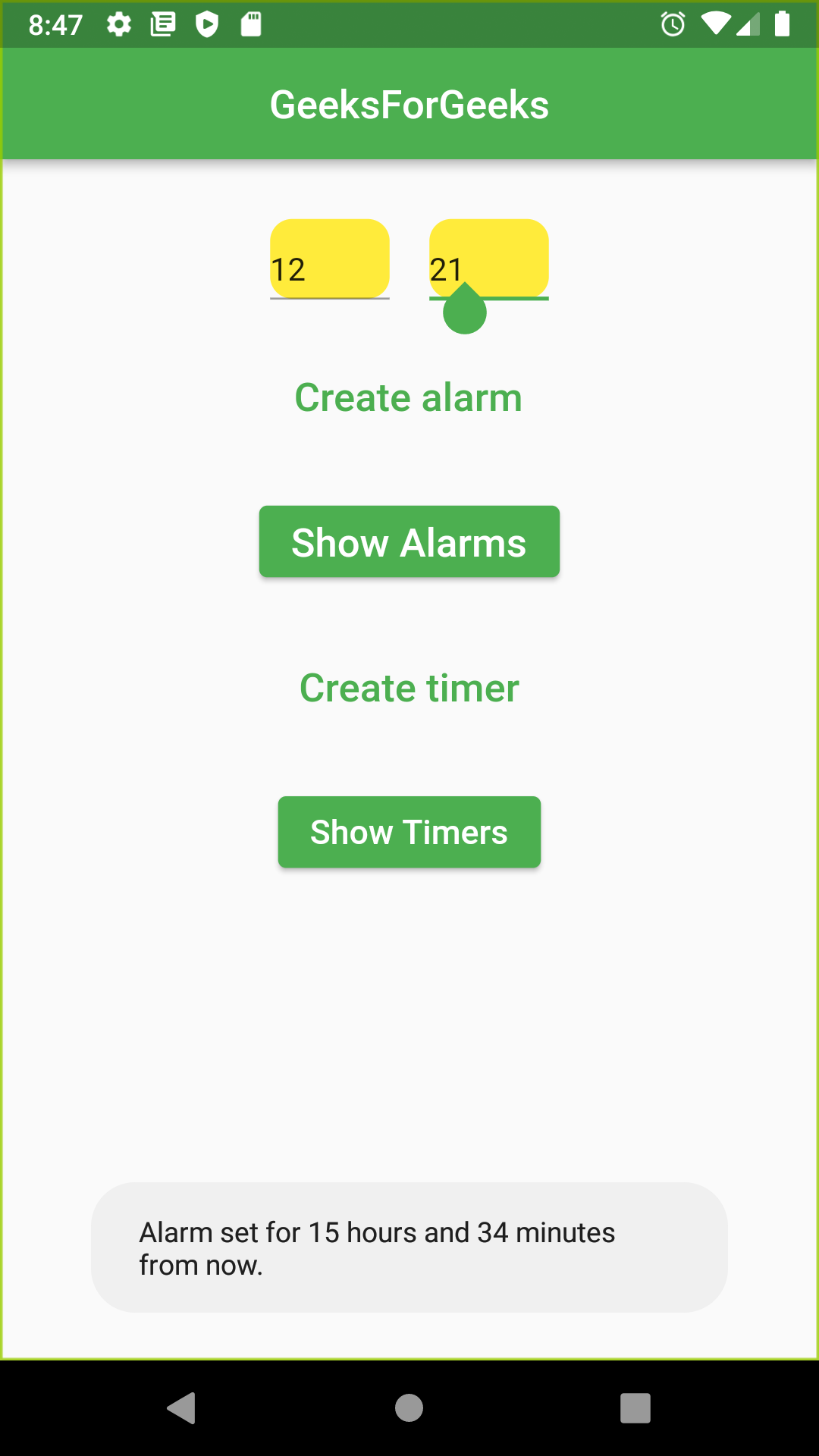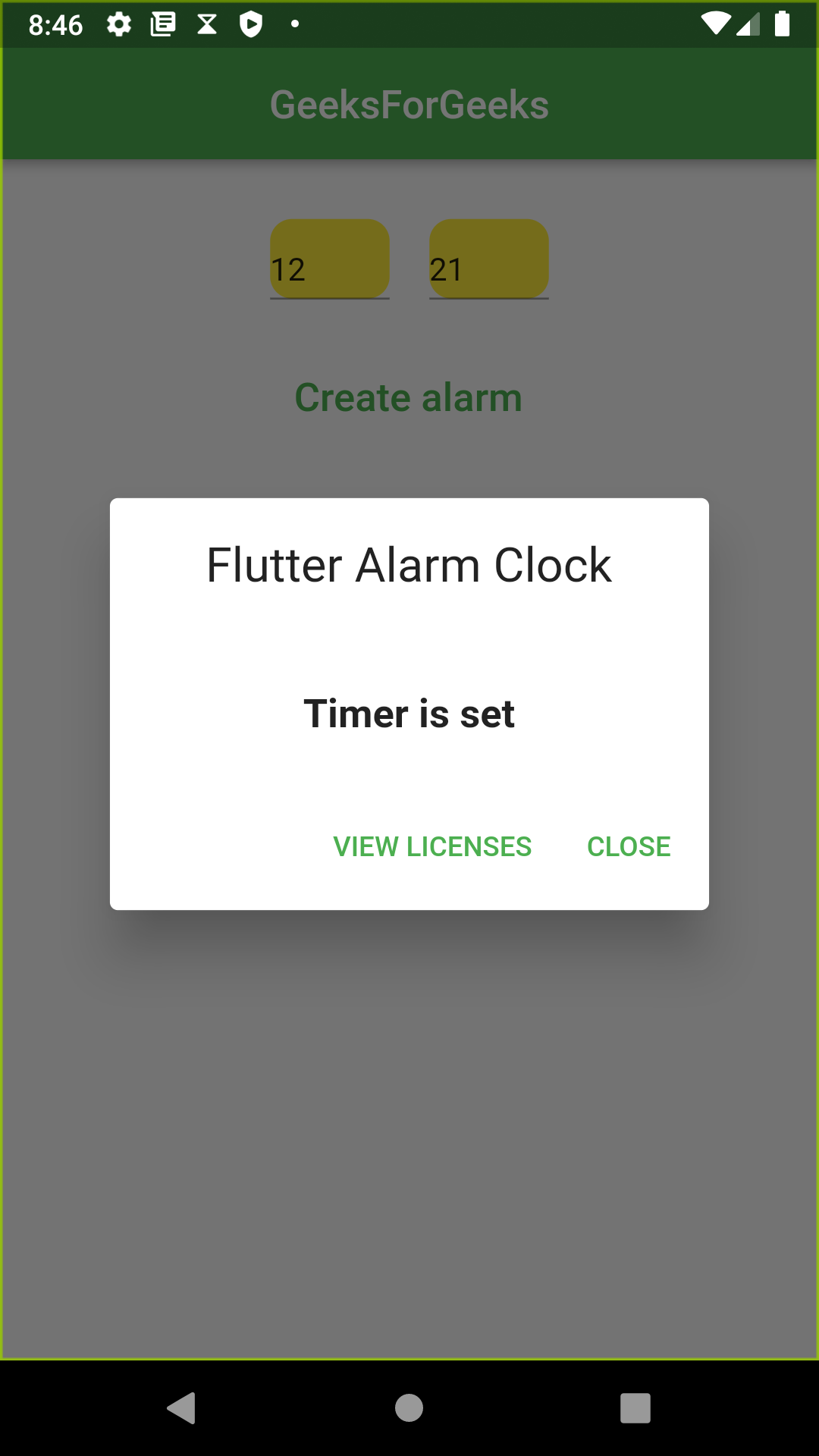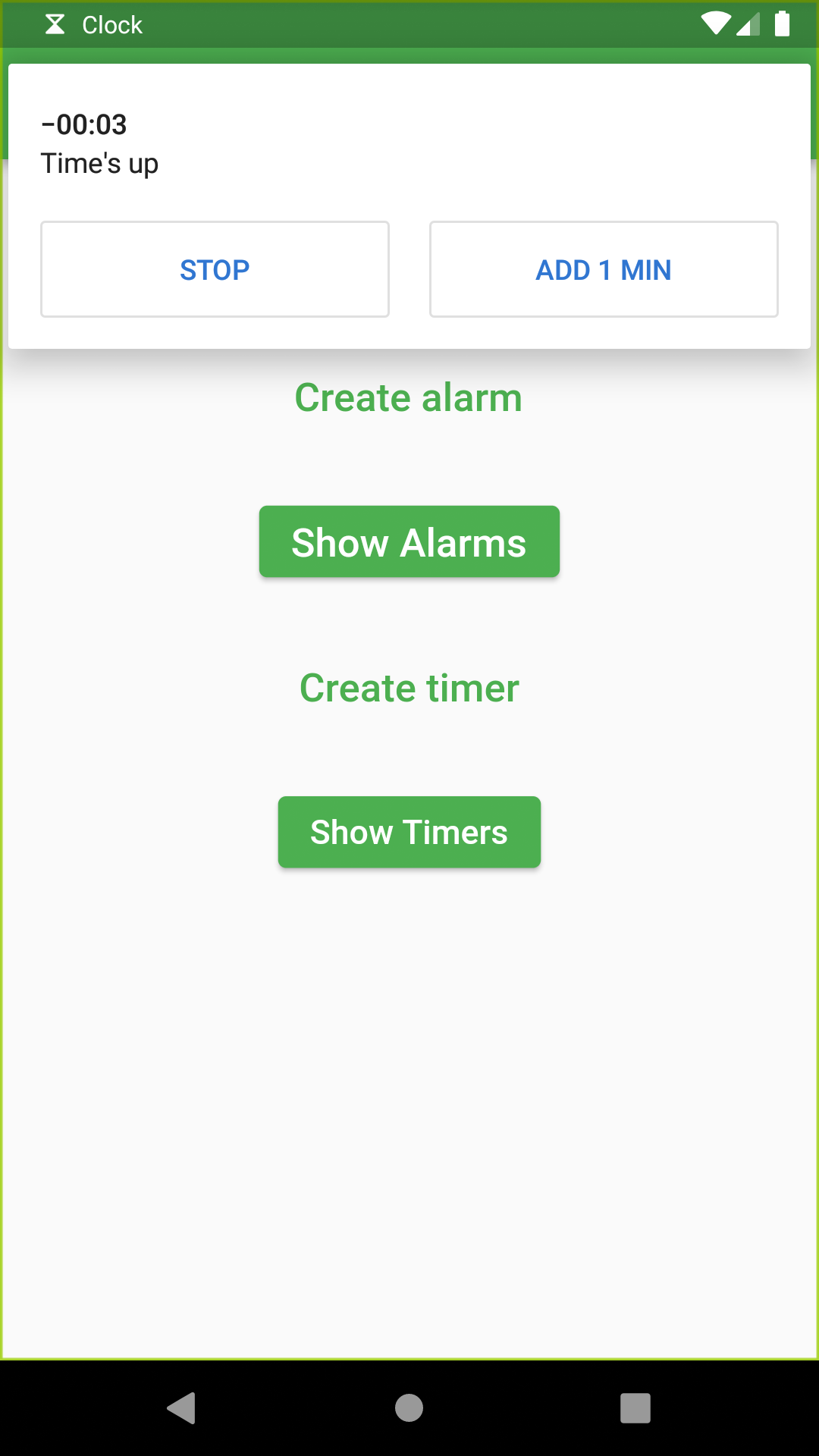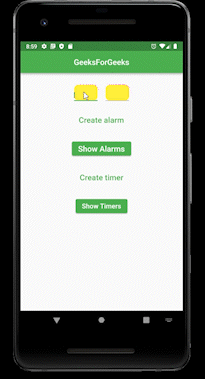# Flutter – Building an Alarm Clock App

• Last Updated : 02 Jan, 2022

Many applications like the Todo app, Exercise App, require alarm clocks to keep track of time in activities. Or if you want to create an alarm clock app for yourself, you will learn this today. If your app contains features like setting the alarm or setting the timer, how you can do this in Flutter? Don’t worry, it’s easy with the flutter_alarm_clock package. In this article, we are going to know the implementation of creating the alarm and timers.

Before we begin with the coding part, first add the following dependency in your pubspec.yaml file.

## Dart

 `dependencies:``  ``flutter_alarm_clock: ^0.0.1`

Now, run pub get to install it.

Step 2: Now, import the library in the file where you want to work with the clock.

## Dart

 `import ``'package:flutter_alarm_clock/flutter_alarm_clock.dart'``;`

### Create an Alarm:

Now, let’s write a code that is going to set an alarm for us. Here, I have wrapped two TextField in a row widgets. We are taking hours and minutes at which the alarm is to be set in input. Created two TextEditingController : hourController and minuteController to take hour and minute in their respective TextField. Then on pressing the button “Create Alarm”, an alarm is being set for that particular time. A snackbar will be shown to show that an alarm has been created with respect to the current time.

## Dart

 `Center(``          ``child: Column(children: [``        ``SizedBox(height: 30),``        ``Row(``          ``mainAxisAlignment: MainAxisAlignment.center,``          ``children: [``            ``Container(``              ``height: 40,``              ``width: 60,``              ``decoration: BoxDecoration(``                  ``shape: BoxShape.rectangle,``                  ``color: Colors.yellow,``                  ``borderRadius: BorderRadius.circular(11)),``              ``child: Center(``                ``child: TextField(``                  ``controller: hourController,``                  ``keyboardType: TextInputType.number,``                ``),``              ``),``            ``),``            ``SizedBox(width: 20),``            ``Container(``              ``height: 40,``              ``width: 60,``              ``decoration: BoxDecoration(``                  ``shape: BoxShape.rectangle,``                  ``color: Colors.yellow,``                  ``borderRadius: BorderRadius.circular(11)),``              ``child: Center(``                ``child: TextField(``                  ``controller: minuteController,``                  ``keyboardType: TextInputType.number,``                ``),``              ``),``            ``),``          ``],``        ``),``        ``Container(``          ``margin: ``const` `EdgeInsets.all(25),``          ``child: TextButton(``            ``child: ``const` `Text(``              ``'Create alarm'``,``              ``style: TextStyle(fontSize: 20.0),``            ``),``            ``onPressed: () {``              ``int` `hour;``              ``int` `minutes;``              ``hour = ``int``.parse(hourController.text);``              ``minutes = ``int``.parse(minuteController.text);``              ``FlutterAlarmClock.createAlarm(hour, minutes);``            ``},``          ``),``        ``),`Then there is another button “Show Alarms” on pressing which will direct to the clock app showing the alarms that have been set.

### Create Timer:

Now, let us set a timer for reminding us that time is up. I am using the minutes taken as input in the above code to set the timer. Note that FlutterAlarmClock.createTimer() takes seconds as inputs. So, we are converting minutes into seconds by multiplying it with 60 to set the timer. When the button “Create Timer” is pressed, the timer is set and a pop-up will show up displaying “Timer is set”. There is an additional button that says “Show Timers”, it will redirect to the clock app to show the timer. When the time is up, a notification by the clock app will be shown that time is up, reset, or add more minutes to the timer.

The code is shown below along with the output.

## Dart

 `Container(``          ``margin: ``const` `EdgeInsets.all(25),``          ``child: TextButton(``              ``child: ``const` `Text(``                ``'Create timer'``,``                ``style: TextStyle(fontSize: 20.0),``              ``),``              ``onPressed: () {``                ``int` `minutes;``                ``minutes = ``int``.parse(minuteController.text);``                ``FlutterAlarmClock.createTimer(minutes*60);``                ``showDialog(``                    ``context: context,``                    ``builder: (context) {``                      ``return` `AboutDialog(``                        ``children: [``                          ``Center(``                            ``child: Text(``"Timer is set"``,``                                ``style: TextStyle(``                                    ``fontSize: 20, fontWeight: FontWeight.bold)),``                          ``)``                        ``],``                      ``);``                    ``});``              ``}),``        ``),``        ``ElevatedButton(``          ``onPressed: () {``            ``FlutterAlarmClock.showTimers();``          ``},``          ``child: Text(``            ``"Show Timers"``,``            ``style: TextStyle(fontSize: 17),``          ``),``  ``)`Complete Source Code:

## Dart

 `import ``'package:flutter/cupertino.dart'``;``import ``'package:flutter/material.dart'``;``import ``'package:flutter_alarm_clock/flutter_alarm_clock.dart'``;`` ` `void` `main() {``  ``runApp(MyApp());``}`` ` `class` `MyApp extends StatelessWidget {``  ``@override``  ``Widget build(BuildContext context) {``    ``return` `MaterialApp(``      ``debugShowCheckedModeBanner: ``false``,``      ``title: ``'Flutter Alarm Clock'``,``      ``theme: ThemeData(``        ``primarySwatch: Colors.green,``      ``),``      ``home: MyHomePage(),``    ``);``  ``}``}`` ` `class` `MyHomePage extends StatefulWidget {``  ``@override``  ``State createState() => _MyHomePageState();``}`` ` `class` `_MyHomePageState extends State {``   ` `  ``// creating text ediiting controller to take hour``  ``// and minute as input``  ``TextEditingController hourController = TextEditingController();``  ``TextEditingController minuteController = TextEditingController();``  ``@override``  ``Widget build(BuildContext context) {``    ``return` `Scaffold(``      ``appBar: AppBar(``        ``title: ``const` `Text(``'GeeksForGeeks'``),``        ``centerTitle: ``true``,``      ``),``      ``body: Center(``          ``child: Column(children: [``        ``SizedBox(height: 30),``        ``Row(``          ``mainAxisAlignment: MainAxisAlignment.center,``          ``children: [``            ``Container(``              ``height: 40,``              ``width: 60,``              ``decoration: BoxDecoration(``                  ``shape: BoxShape.rectangle,``                  ``color: Colors.yellow,``                  ``borderRadius: BorderRadius.circular(11)),``              ``child: Center(``                ``child: TextField(``                  ``controller: hourController,``                  ``keyboardType: TextInputType.number,``                ``),``              ``),``            ``),``            ``SizedBox(width: 20),``            ``Container(``              ``height: 40,``              ``width: 60,``              ``decoration: BoxDecoration(``                  ``shape: BoxShape.rectangle,``                  ``color: Colors.yellow,``                  ``borderRadius: BorderRadius.circular(11)),``              ``child: Center(``                ``child: TextField(``                  ``controller: minuteController,``                  ``keyboardType: TextInputType.number,``                ``),``              ``),``            ``),``          ``],``        ``),``        ``Container(``          ``margin: ``const` `EdgeInsets.all(25),``          ``child: TextButton(``            ``child: ``const` `Text(``              ``'Create alarm'``,``              ``style: TextStyle(fontSize: 20.0),``            ``),``            ``onPressed: () {``              ``int` `hour;``              ``int` `minutes;``              ``hour = ``int``.parse(hourController.text);``              ``minutes = ``int``.parse(minuteController.text);``               ` `              ``// creating alarm after converting hour ``              ``// and minute into integer``              ``FlutterAlarmClock.createAlarm(hour, minutes);``            ``},``          ``),``        ``),``        ``ElevatedButton(``          ``onPressed: () {``             ` `            ``// show alarm``            ``FlutterAlarmClock.showAlarms();``          ``},``          ``child: ``const` `Text(``            ``'Show Alarms'``,``            ``style: TextStyle(fontSize: 20.0),``          ``),``        ``),``        ``Container(``          ``margin: ``const` `EdgeInsets.all(25),``          ``child: TextButton(``              ``child: ``const` `Text(``                ``'Create timer'``,``                ``style: TextStyle(fontSize: 20.0),``              ``),``              ``onPressed: () {``                ``int` `minutes;``                ``minutes = ``int``.parse(minuteController.text);``                 ` `                ``// create timer``                ``FlutterAlarmClock.createTimer(minutes);``                ``showDialog(``                    ``context: context,``                    ``builder: (context) {``                      ``return` `AboutDialog(``                        ``children: [``                          ``Center(``                            ``child: Text(``"Timer is set"``,``                                ``style: TextStyle(``                                    ``fontSize: 20, fontWeight: FontWeight.bold)),``                          ``)``                        ``],``                      ``);``                    ``});``              ``}),``        ``),``        ``ElevatedButton(``          ``onPressed: () {``             ` `            ``// show timers``            ``FlutterAlarmClock.showTimers();``          ``},``          ``child: Text(``            ``"Show Timers"``,``            ``style: TextStyle(fontSize: 17),``          ``),``        ``)``      ``])),``    ``);``  ``}``}`My Personal Notes arrow_drop_up